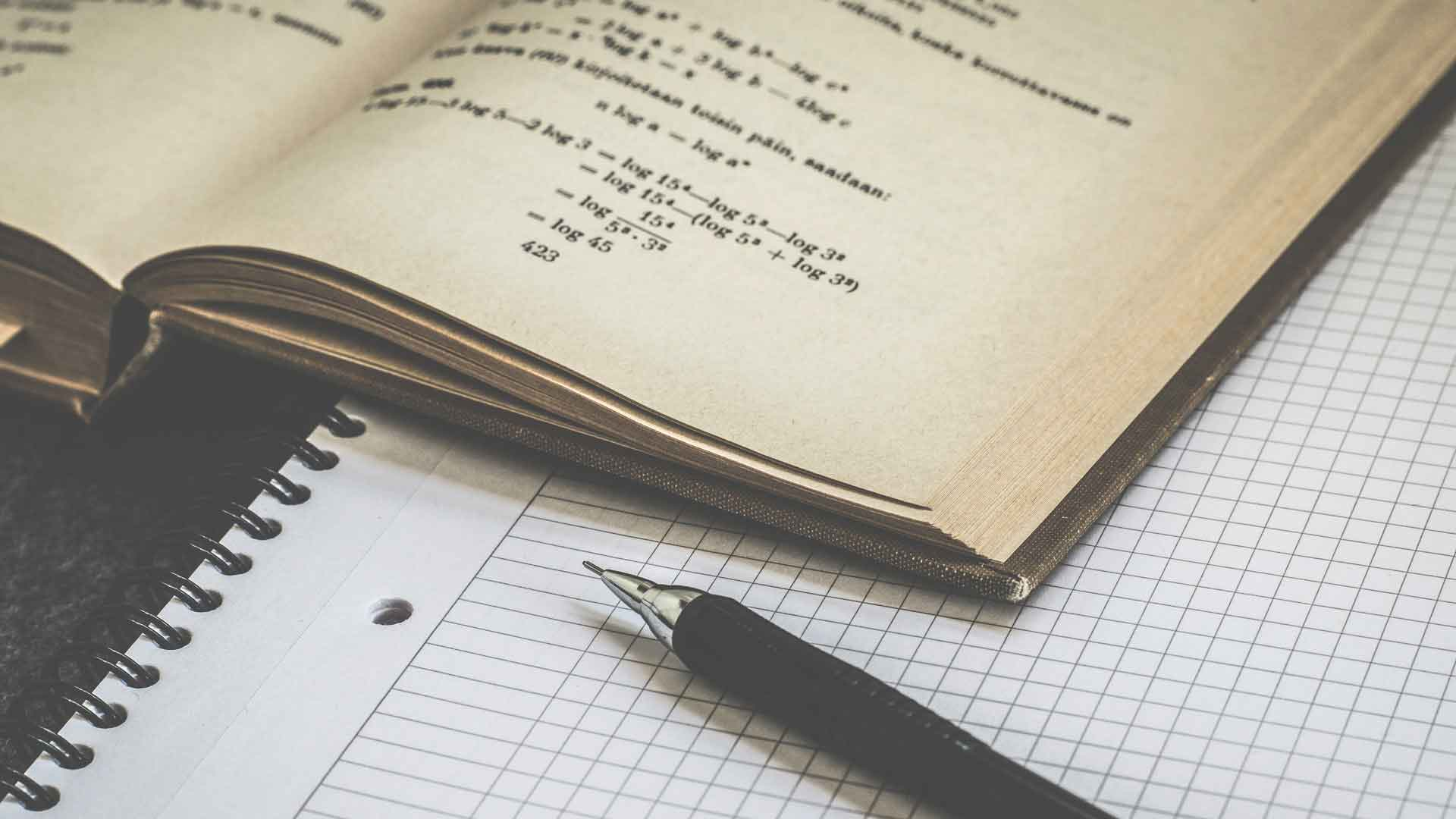Fact checked# How Can I Score Well on the GED® Math Test?

The best way to score well on the GED® math test is to study the four content areas tested, take practice tests to help you...
Mathematics3 minutesPassing the General Education Development® (GED®) test gives a person the equivalent of a high school diploma. The math test is one of five sections of the exam. To score well on the GED® math test, you need a sound understanding of the concepts tested in each of the four content areas covered in this section. Studying and practicing to improve test-taking skills will also help you achieve a higher score.

You need to score at least 410 out of 800 total points to pass the GED® math test. This equates to getting about 30 to 33 questions correct out of the 50 questions in this section. Passing the entire exam requires an average of 450 on each of the five sections, so a higher score on the math section helps your overall score as well.

Consider taking a practice test before you start studying so you can identify which math concepts require more focus. The GED® math test is comprised of two sections covering four content areas, each of which comprises 20 to 30 percent of the math test, or 10 to 15 questions, so you will want to do well in all areas. Choosing an online test that shows your scores for each area so you know which concepts you need to study can be especially helpful.

The number operations and number sense content area covers basic arithmetic operations using various methods of representing numbers, such as fractions, decimals, and percentages. Measurement and geometry questions test basic principles of geometry and measurement concepts, such as converting units and calculating area and volume. The data, analysis, statistics, and probability section tests your ability to interpret and represent data in graphs, evaluate arguments, make predictions based on data, and calculate basic statistical parameters such as mean and mode. Questions on algebra, functions, and patterns cover basic algebra, such as solving and graphing equations with variables.

Study for each of the content areas, but focus on the areas where your scores were weakest on the practice test. You may want to purchase a test preparation book to help you study. High school algebra and geometry textbooks can also help. The American Council on Education, which develops the GED® test, sells test preparation materials, as do several other publishers.

Experts generally recommend practicing with test questions, which commonly are found in test preparation books. The GED® math test has two sections of 25 questions each. You can use a calculator for the first section, but no calculator is allowed for the second section, only a formula sheet. Practice solving questions without a calculator using the formula sheet so you will be comfortable doing it on test day. Most questions are multiple choice, but each section also has five questions that require you to graph the answer on a coordinate system, so practice graphing answers as well.

Use strategies on the day of the test to help you narrow down answers and get through all the GED® math test questions in the allotted time. Read the question and all the answers first and eliminate any answers that are obviously wrong. Estimate an answer before performing complex calculations and see if you can pick an answer based on the estimate. If a question has units, double-check which units the answer should be in and eliminate any answers with different units.

Do you need one-on-one GED math tutoring? Get matched with an online math tutor by speaking with our academic advisor today!### Get started with a custom-matched tutor for your child.